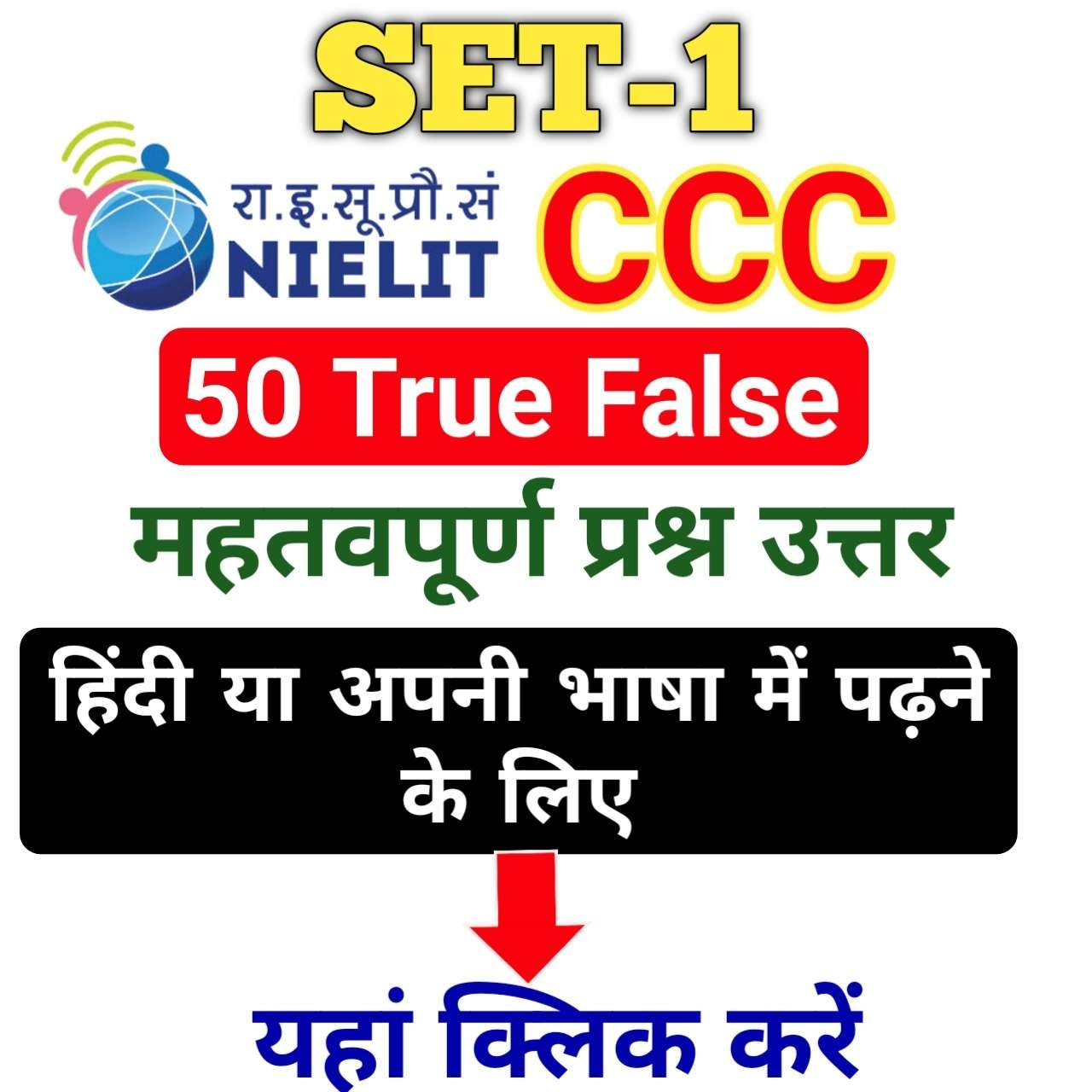# Amit Tech Engineering: SarkariResult.Com Sarkari Naukri Latest Jobs Online Form at Sarkari Results 2023

www.ateo.in India No 1 Education Portal

CCC True False Question Answer PDF English/Hindi1. TRUE
2. FALSE

1. TRUE

1. TRUE
2. FALSE

1. TRUE

1. TRUE
2. FALSE

1. TRUE

1. TRUE
2. FALSE

1. FALSE

1. TRUE
2. FALSE

1. FALSE

1. TRUE
2. FALSE

1. TRUE

1. TRUE
2. FALSE

1. TRUE

1. TRUE
2. FALSE

1. TRUE

1. TRUE
2. FALSE

1. TRUE

1. TRUE
2. FALSE

1. TRUE

1. TRUE
2. FALSE

1. FALSE

1. TRUE
2. FALSE

1. TRUE

1. TRUE
2. FALSE

1. TRUE

1. TRUE
2. FALSE

1. TRUE

1. TRUE
2. FALSE

1. TRUE

1. TRUE
2. FALSE

1. TRUE

1. TRUE
2. FALSE

1. TRUE

1. TRUE
2. FALSE

1. TRUE

1. TRUE
2. FALSE

1. TRUE

1. TRUE
2. FALSE

1. TRUE

1. TRUE
2. FALSE

1. TRUE

1. TRUE
2. FALSE

1. FALSE

1. TRUE
2. FALSE

1. TRUE

1. TRUE
2. FALSE

1. TRUE

1. TRUE
2. FALSE

1. FALSE

1. TRUE
2. FALSE

1. TRUE

1. TRUE
2. FALSE

1. FALSE

1. TRUE
2. FALSE

1. TRUE

1. TRUE
2. FALSE

1. FALSE

1. TRUE
2. FALSE

1. FALSE

1. TRUE
2. FALSE

1. TRUE

1. TRUE
2. FALSE

1. TRUE

1. TRUE
2. FALSE

1. TRUE

1. TRUE
2. FALSE

1. TRUE

1. TRUE
2. FALSE

1. TRUE

1. TRUE
2. FALSE

1. TRUE

1. TRUE
2. FALSE

1. FALSE

1. TRUE
2. FALSE

1. TRUE

1. TRUE
2. FALSE

1. TRUE

1. TRUE
2. FALSE

1. FALSE

1. TRUE
2. FALSE

1. FALSE

1. TRUE
2. FALSE

1. TRUE

1. TRUE
2. FALSE

1. TRUE

1. TRUE
2. FALSE

1. TRUE

1. TRUE
2. FALSE

1. TRUE

1. TRUE
2. FALSE

1. TRUE

1. TRUE
2. FALSE

1. TRUE

1. TRUE
2. FALSE

1. TRUE

1. TRUE
2. FALSE

1. TRUE

1. TRUE
2. FALSE

1. TRUE

Scroll to Top